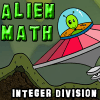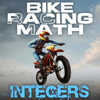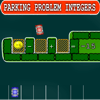Custom Search

# Find Our Cool Math Games to Play by Category:

### 7.NS.A.2.b

Understand that integers can be divided, provided that the divisor is not zero, and every quotient of integers (with non-zero divisor) is a rational number. If p and q are integers, then -(p/q) = (-p)/q = p/(-q). Interpret quotients of rational numbers by describing real-world contexts.CCSS: 7.NS.A.2.b
Take an alien flying saucer for a spin and collect numbered fuel pods that answer the integer division problems.  Collecting fuel pods keep your saucer flying.  Try flying as long as you can.CCSS: 7.NS.A.1.b, 7.NS.A.1.c, 7.NS.A.2.a, 7.NS.A.2.b
Race your motorcycle to the finish line.  Race against some tough competitors and try and beat them all by answering problems with positive and negative numbers.CCSS: 7.NS.A.1.b, 7.NS.A.1.c, 7.NS.A.2.a, 7.NS.A.2.b
Pick up numbers, both positive and negative, and drop them in the problem parking spots to build a valid equation equal to the target number of each level.  Choose to play the addition/subtraction version or the multiplication/division version.